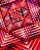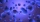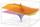# Geometric sequence 3

In geometric sequence is a8 = 312500; a11= 39062500; sn=1953124. Calculate the first item a1, quotient q, and n - number of members by their sum s_n.

a1 =  4
q =  5
n =  9

### Step-by-step explanation:Did you find an error or inaccuracy? Feel free to write us. Thank you!Tips to related online calculators
Do you have a system of equations and looking for calculator system of linear equations?

## Related math problems and questions:

• Geometric sequenceIn the geometric sequence is a4 = 20 a9 = -160. Calculate the first member a1 and quotient q.
• Geometric progressionIn geometric progression, a1 = 7, q = 5. Find the condition for n to sum first n members is sn≤217.
• Geometric sequence 4It is given geometric sequence a3 = 7 and a12 = 3. Calculate s23 (= sum of the first 23 members of the sequence).
• Five membersWrite first 5 members geometric sequence and determine whether it is increasing or decreasing: a1 = 3 q = -2
• Geometric sequence 5About members of geometric sequence we know: ? ? Calculate a1 (first member) and q (common ratio or q-coefficient)
• Five elementThe geometric sequence is given by quotient q = 1/2 and the sum of the first six members S6 = 63. Find the fifth element a5.
• Three members GPThe sum of three numbers in GP (geometric progression) is 21 and the sum of their squares is 189. Find the numbers.
• InsertInsert five numbers between 8 and 27 such numbers that, with two given ones, they form the first seven members of the geometric sequence.
• MicroorganismsThe first generation of micro-organisms has a population of 13500 members. Each next generation is 11/10 times the previous one. Find out how many generations will reach at least three times members of the first generation.
• Sequence - 5 membersWrite first five members of the sequence ?
• SequenceCalculate what member of the sequence specified by (5n-2+15) has value 20.
• SequenceWrite the first 7 members of an arithmetic sequence: a1=-3, d=6.
• GP - three membersThe second and third of a geometric progression are 24 and 12(c+1), respectively, given that the sum of the first three terms of progression is 76. determine the value of c.
• Sum-logThe sum of two numbers is 32, the sum of their logarithms (base 10) is 2.2. Determine these numbers.
• Sequences AP + GPThe three numbers that make up the arithmetic sequence have the sum of 30. If we subtract from the first 5, from the second 4 and keep the third, we get the geometric sequence. Find AP and GP members.
• SequenceIn the arithmetic sequence is a1=-1, d=4. Which member is equal to the number 203?
• GP - 8 itemsDetermine the first eight members of a geometric progression if a9=512, q=2